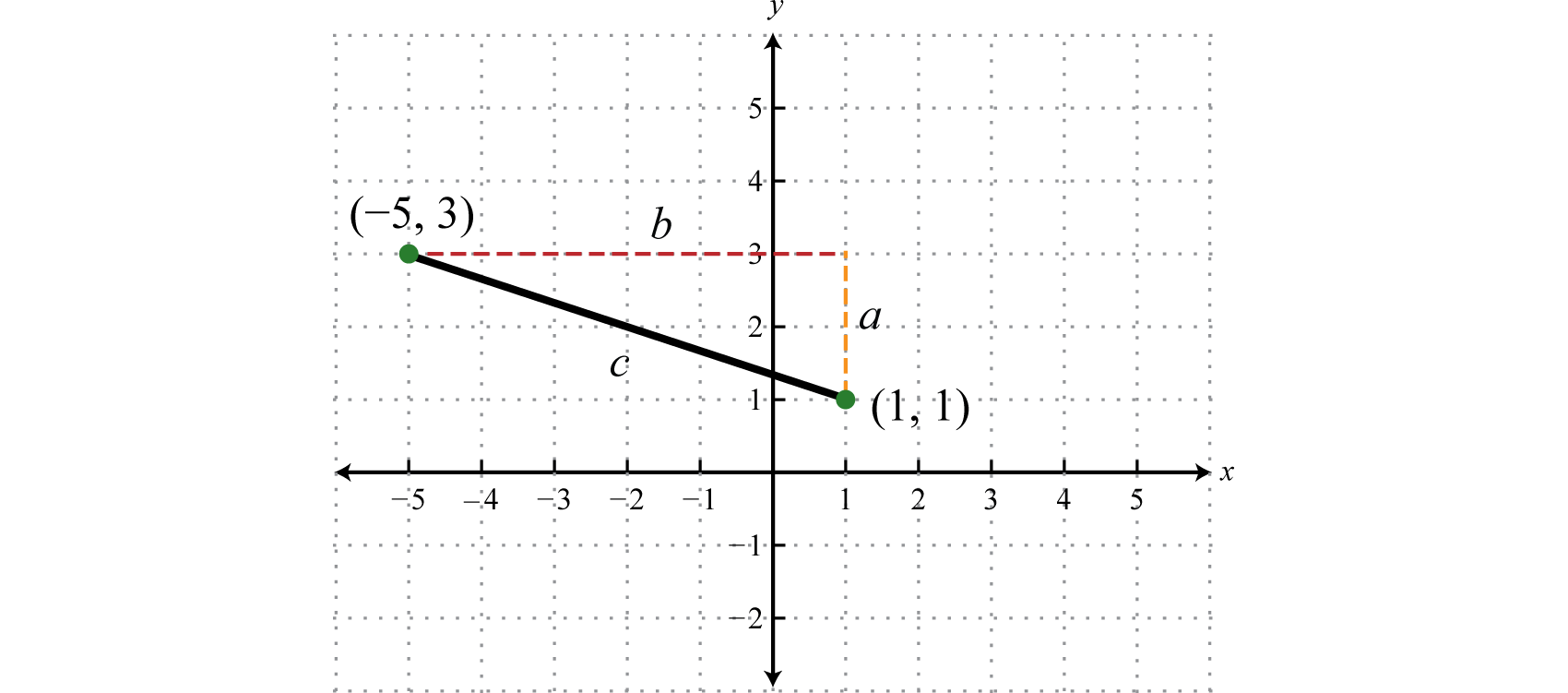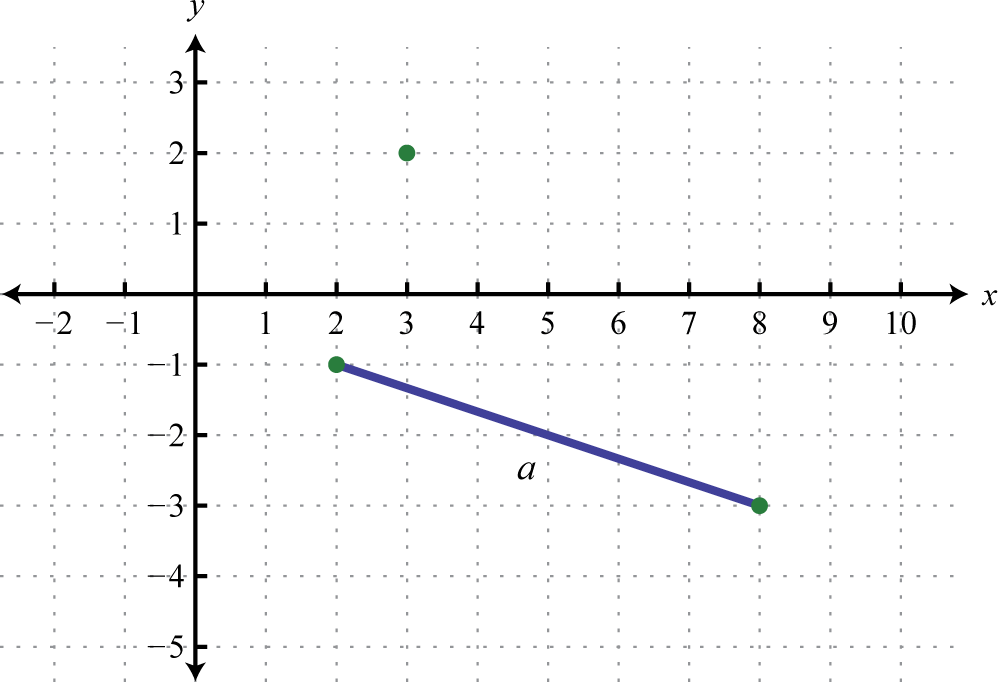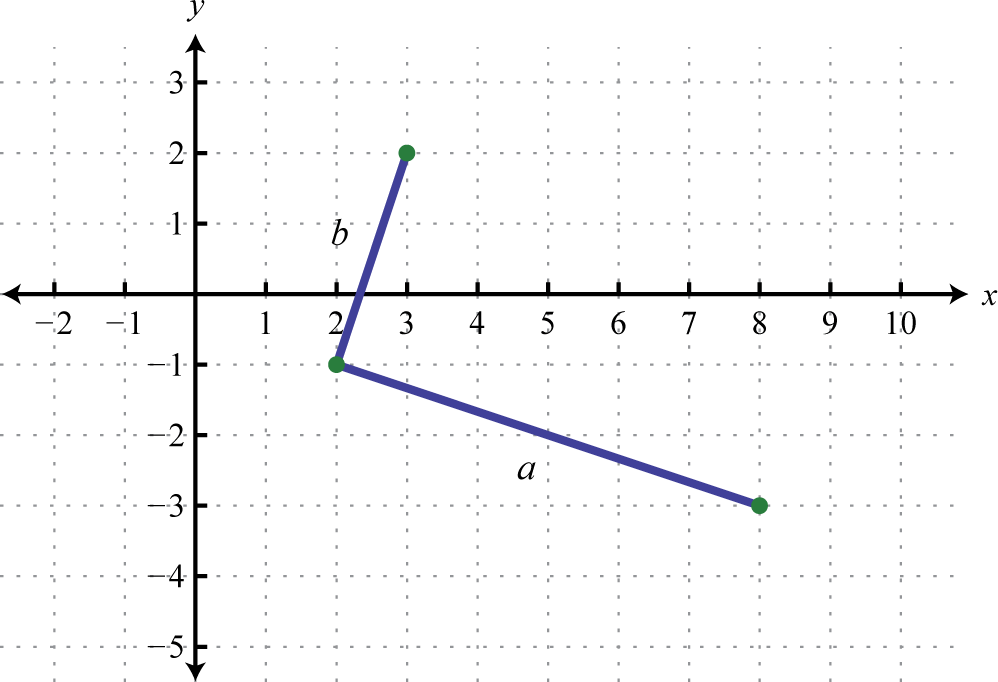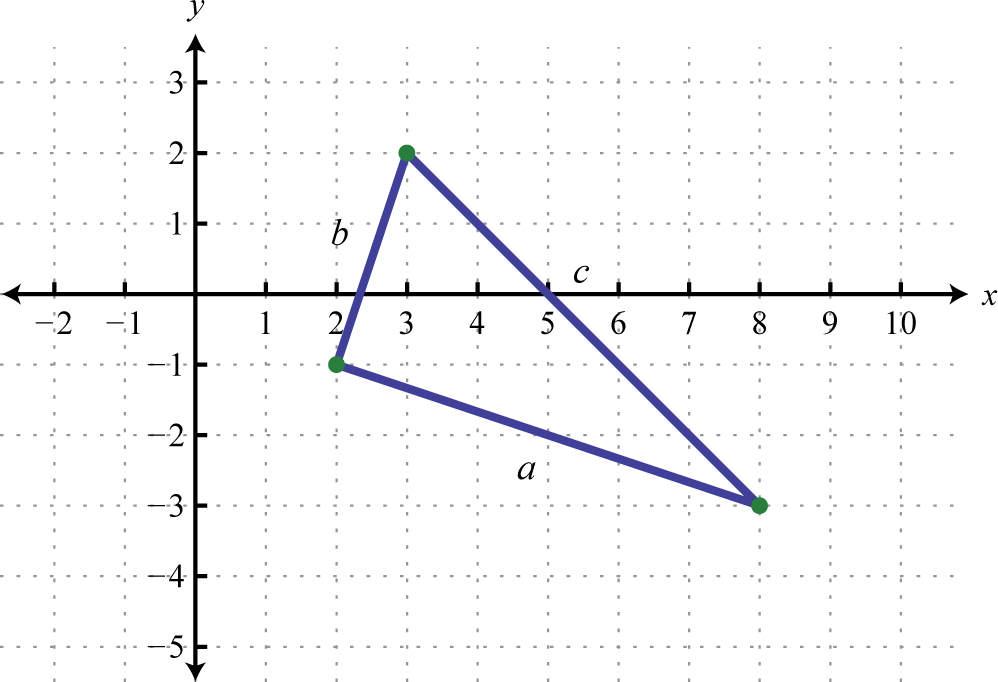Has this book helped you? Consider passing it on:
Creative Commons supports free culture from music to education. Their licenses helped make this book available to you.
DonorsChoose.org helps people like you help teachers fund their classroom projects, from art supplies to books to calculators.

### Learning Objectives

1. Simplify radical expressions using the product and quotient rule for radicals.

An algebraic expression that contains radicals is called a radical expressionAn algebraic expression that contains radicals.. We use the product and quotient rules to simplify them.

### Example 1

Simplify: $27x33.$

Solution:

Use the fact that $ann=a$ when n is odd.

$27x33=33⋅x33Apply the product rule for radicals.=333⋅x33Simplify.=3⋅x=3x$

Answer: $3x$

### Example 2

Simplify: $16y44.$

Solution:

Use the fact that $ann=|a|$ when n is even.

$16y44=24y44Apply the product rule for radicals.=244⋅y44Simplify.=2⋅|y|=2|y|$

Since y is a variable, it may represent a negative number. Thus we need to ensure that the result is positive by including the absolute value.

Answer: $2|y|$

### Important Note

Typically, at this point in algebra we note that all variables are assumed to be positive. If this is the case, then y in the previous example is positive and the absolute value operator is not needed. The example can be simplified as follows.

$16y44=24y44 =244⋅y44=2y$

In this section, we will assume that all variables are positive. This allows us to focus on calculating nth roots without the technicalities associated with the principal nth root problem. For this reason, we will use the following property for the rest of the section,

$ann=a, if a≥0 nth root$

When simplifying radical expressions, look for factors with powers that match the index.

### Example 3

Simplify: $12x6y3.$

Solution:

Begin by determining the square factors of 12, $x6$, and $y3.$

$12=22⋅3x6=(x3)2 y3=y2⋅y } Square factors$

Make these substitutions, and then apply the product rule for radicals and simplify.

$12x6y3=22⋅3⋅(x3)2⋅y2⋅yApply the product rule for radicals.=22⋅(x3)2⋅y2⋅3ySimplify.=2 ⋅ x3 ⋅ y ⋅3y=2x3y3y$

Answer: $2x3y3y$

### Example 4

Simplify: $18a5b8$.

Solution:

Begin by determining the square factors of 18, $a5$, and $b8.$

$18=2⋅32a5=a2⋅a2⋅a=(a2)2⋅a b8=b4⋅b4=(b4)2 } Square factors$

Make these substitutions, apply the product and quotient rules for radicals, and then simplify.

$18a5b8=2⋅32⋅(a2)2⋅a(b4)2Apply the product and quotient rule for radicals.=32⋅(a2)2⋅2a(b4)2Simplify.= 3a22ab4$

Answer: $3a22ab4$

### Example 5

Simplify: $80x5y73.$

Solution:

Begin by determining the cubic factors of 80, $x5$, and $y7.$

$80=24⋅5=23⋅2⋅5x5=x3⋅x2y7=y6⋅y=(y2)3⋅y } Cubic factors$

Make these substitutions, and then apply the product rule for radicals and simplify.

$80x5y73=23⋅2⋅5⋅x3⋅x2⋅(y2)3⋅y3 =233⋅x33⋅(y2)33⋅2⋅5⋅x2⋅y3=2⋅xy2⋅10x2y3=2xy2 10x2y3$

Answer: $2xy210x2y3$

### Example 6

Simplify $9x6y3z93$.

Solution:

The coefficient $9=32$, and thus does not have any perfect cube factors. It will be left as the only remaining radicand because all of the other factors are cubes, as illustrated below:

$x6=(x2)3y3=(y)3z9=(z3)3 } Cubic factors$

Replace the variables with these equivalents, apply the product and quotient rules for radicals, and then simplify.

$9x6y3z93=9⋅(x2)3y3⋅(z3)33=93⋅(x2)33y33⋅(z3)33=93⋅x2y⋅z3=x2 93yz3$

Answer: $x293yz3$

### Example 7

Simplify: $81a4b54.$

Solution:

Determine all factors that can be written as perfect powers of 4. Here, it is important to see that $b5=b4⋅b.$ Hence the factor $b$ will be left inside the radical.

$81a4b54=34⋅a4⋅b4⋅b4=344⋅a44⋅b44⋅b4=3⋅a⋅b⋅b4=3abb4$

Answer: $3abb4$

### Example 8

Simplify: $−32x3y6z55.$

Solution:

Notice that the variable factor x cannot be written as a power of 5 and thus will be left inside the radical. In addition, $y6=y5⋅y$; the factor y will be left inside the radical as well.

$−32x3y6z55=(−2)5⋅x3⋅y5⋅y⋅z55=(−2)55⋅y55⋅z55⋅x3⋅y5=−2⋅y⋅z⋅x3⋅y5=−2yzx3y5$

Answer: $−2yzx3y5$

Tip: To simplify finding an nth root, divide the powers by the index.

$a6=a3, which is a6÷2=a3b63=b2, which is b6÷3=b2c66=c , which is c6÷6=c1$

If the index does not divide into the power evenly, then we can use the quotient and remainder to simplify. For example,

$a5=a2⋅a, which is a5÷2=a2 r 1b53=b⋅b23, which is b5÷3=b1 r 2c145=c2⋅c45, which is c14÷5=c2 r 4$

The quotient is the exponent of the factor outside of the radical, and the remainder is the exponent of the factor left inside the radical.

Try this! Simplify: $162a7b5c43.$

Answer: $3a2bc6ab2c3$

Formulas often consist of radical expressions. For example, the period of a pendulum, or the time it takes a pendulum to swing from one side to the other and back, depends on its length according to the following formula.

$T=2πL32$

Here T represents the period in seconds and L represents the length in feet of the pendulum.

### Example 9

If the length of a pendulum measures $112$ feet, then calculate the period rounded to the nearest tenth of a second.

Solution:

Substitute $112=32$ for L and then simplify.

$T=2πL32=2π3232=2π32⋅132Apply the quotient rule for radicals.=2π364Simplify.=2π38 =π34≈1.36$

Answer: The period is approximately 1.36 seconds.

Frequently you need to calculate the distance between two points in a plane. To do this, form a right triangle using the two points as vertices of the triangle and then apply the Pythagorean theorem. Recall that the Pythagorean theorem states that if given any right triangle with legs measuring a and b units, then the square of the measure of the hypotenuse c is equal to the sum of the squares of the legs: $a2+b2=c2.$ In other words, the hypotenuse of any right triangle is equal to the square root of the sum of the squares of its legs.### Example 10

Find the distance between (−5, 3) and (1, 1).

Solution:

Form a right triangle by drawing horizontal and vertical lines though the two points. This creates a right triangle as shown below:The length of leg b is calculated by finding the distance between the x-values of the given points, and the length of leg a is calculated by finding the distance between the given y-values.

$a=3−1=2 unitsb=1−(−5)=1+5=6 units$

Next, use the Pythagorean theorem to find the length of the hypotenuse.

$c=22+62=4+36=40=4⋅10=210 units$

Answer: The distance between the two points is $210$ units.

Generalize this process to produce a formula that can be used to algebraically calculate the distance between any two given points.Given two points, $( x1, y1)$ and $( x2, y2)$, the distance, d, between them is given by the distance formulaGiven two points $(x1, y1)$ and $(x2, y2)$, calculate the distance d between them using the formula $d=(x2−x1)2+(y2−y1)2.$, $d=(x2−x1)2+(y2−y1)2.$

### Example 11

Calculate the distance between (−4, 7) and (2, 1).

Solution:

Use the distance formula with the following points.

$( x1, y1) ( x2, y2)(−4,7)(2, 1)$

It is a good practice to include the formula in its general form before substituting values for the variables; this improves readability and reduces the probability of making errors.

$d=(x2−x1)2+(y2−y1)2=(2−(−4))2+(1−7)2=(2+4)2+(1−7)2=(6)2+(−6)2=36+36=72=36⋅2=62$

Answer: The distance between the two points is $62$ units.

### Example 12

Do the three points (2, −1), (3, 2), and (8, −3) form a right triangle?

Solution:

The Pythagorean theorem states that having side lengths that satisfy the property $a2+b2=c2$ is a necessary and sufficient condition of right triangles. In other words, if you can show that the sum of the squares of the leg lengths of the triangle is equal to the square of the length of the hypotenuse, then the triangle must be a right triangle. First, calculate the length of each side using the distance formula.

Geometry

CalculationPoints: (2, −1) and (8, −3)

$a=(8−2)2+[−3−(−1)]2=(6)2+(−3+1)2=36+(−2)2=36+4=40=210$Points: (2, −1) and (3, 2)

$b=(3−2)2+[2−(−1)]2=(1)2+(2+1)2=1+(3)2=1+9=10$Points: (3, 2) and (8, −3)

$c=(8−3)2+(−3−2)2=(5)2+(−5)2=25+25=50=52$

Now we check to see if $a2+b2=c2.$

$a2+b2=c2(210)2+(10)2=(52)24(10)2+(10)2=25(2)24⋅10+10=25⋅250=50 ✓$

Answer: Yes, the three points form a right triangle.

Try this! The speed of a vehicle before the brakes were applied can be estimated by the length of the skid marks left on the road. On wet concrete, the speed v in miles per hour can be estimated by the formula $v=23d$, where d represents the length of the skid marks in feet. Estimate the speed of a vehicle before applying the brakes if the skid marks left behind measure 27 feet. Round to the nearest mile per hour.

### Key Takeaways

• To simplify a radical expression, look for factors of the radicand with powers that match the index. If found, they can be simplified by applying the product and quotient rules for radicals, as well as the property $ann=a$, where a is nonnegative.
• A radical expression is simplified if its radicand does not contain any factors that can be written as perfect powers of the index.
• We typically assume that all variable expressions within the radical are nonnegative. This allows us to focus on simplifying radicals without the technical issues associated with the principal nth root. If this assumption is not made, we will ensure a positive result by using absolute values when simplifying radicals with even indices.

### Part A: Simplifying Radical Expressions

Assume that the variable could represent any real number and then simplify.

1. $9x2$

2. $16y2$

3. $8y33$

4. $125a33$

5. $64x44$

6. $81y44$

7. $36a4$

8. $100a8$

9. $4a6$

10. $a10$

11. $18a4b5$

12. $48a5b3$

13. $128x6y86$

14. $a6b7c86$

15. $(5x−4)2$

16. $(3x−5)4$

17. $x2−6x+9$

18. $x2−10x+25$

19. $4x2+12x+9$

20. $9x2+6x+1$

Simplify. (Assume all variable expressions represent positive numbers.)

1. $49a2$

2. $64b2$

3. $x2y2$

4. $25x2y2z2$

5. $180x3$

6. $150y3$

7. $49a3b2$

8. $4a4b3c$

9. $45x5y3$

10. $50x6y4$

11. $64r2s6t5$

12. $144r8s6t2$

13. $(x+1)2$

14. $(2x+3)2$

15. $4(3x−1)2$

16. $9(2x+3)2$

17. $9x325y2$
18. $4x59y4$
19. $m736n4$
20. $147m9n6$
21. $2r2s525t4$
22. $36r5s2t6$
23. $27a33$

24. $125b33$

25. $250x4y33$

26. $162a3b53$

27. $64x3y6z93$

28. $216x12y33$

29. $8x3y43$

30. $27x5y33$

31. $a4b5c63$

32. $a7b5c33$

33. $8x427y33$
34. $x5125y63$
35. $360r5s12t133$

36. $540r3s2t93$

37. $81x44$

38. $x4y44$

39. $16x4y84$

40. $81x12y44$

41. $a4b5c64$

42. $54a6c84$

43. $128x64$

44. $243y74$

45. $32m10n55$
46. $37m9n105$
47. $−34x2$

48. $79y2$

49. $−5x4x2y$

50. $−3y16x3y2$

51. $12aba5b3$

52. $6a2b9a7b2$

53. $2x8x63$

54. $−5x227x33$

55. $2ab−8a4b53$

56. $5a2b−27a3b33$

Rewrite the following as a radical expression with coefficient 1.

1. $3x6x$

2. $5y5y$

3. $ab10a$

4. $2ab2a$

5. $m2nmn$

6. $2m2n33n$

7. $2x3x3$

8. $3yy23$

9. $2y24y4$

10. $x2y9xy25$

### Part B: Formulas Involving Radicals

The period T in seconds of a pendulum is given by the formula $T=2πL32$ where L represents the length in feet of the pendulum. Calculate the period, given each of the following lengths. Give the exact value and the approximate value rounded to the nearest tenth of a second.

1. 8 feet

2. 32 feet

3. $12$ foot

4. $18$ foot

The time t in seconds an object is in free fall is given by the formula $t=s4$ where s represents the distance in feet the object has fallen. Calculate the time it takes an object to fall, given each of the following distances. Give the exact value and the approximate value rounded to the nearest tenth of a second.

1. 48 feet

2. 80 feet

3. 192 feet

4. 288 feet

5. The speed of a vehicle before the brakes were applied can be estimated by the length of the skid marks left on the road. On dry pavement, the speed v in miles per hour can be estimated by the formula $v=26d$, where d represents the length of the skid marks in feet. Estimate the speed of a vehicle before applying the brakes on dry pavement if the skid marks left behind measure 27 feet. Round to the nearest mile per hour.

6. The radius r of a sphere can be calculated using the formula $r=6π2V32π$, where V represents the sphere’s volume. What is the radius of a sphere if the volume is $36π$ cubic centimeters?

Given the function find the y-intercept

1. $f(x)=x+12$

2. $f(x)=x+8−3$

3. $f(x)=x−83$

4. $f(x)=x+273$

5. $f(x)=x+163$

6. $f(x)=x+33−1$

Use the distance formula to calculate the distance between the given two points.

1. (5, −7) and (3, −8)

2. (−9, 7) and (−8, 4)

3. (−3, −4) and (3, −6)

4. (−5, −2) and (1, −6)

5. (−1, 1) and (−4, 10)

6. (8, −3) and (2, −12)

7. (0, −6) and (−3, 0)

8. (0, 0) and (8, −4)

9. $(12,−12)$ and $(−1,32)$

10. $(−13,2)$ and $(53,−23)$

Determine whether or not the three points form a right triangle. Use the Pythagorean theorem to justify your answer.

1. (2,−1), (−1,2), and (6,3)

2. (−5,2), (−1, −2), and (−2,5)

3. (−5,0), (0,3), and (6,−1)

4. (−4,−1), (−2,5), and (7,2)

5. (1,−2), (2,3), and (−3,4)

6. (−2,1), (−1,−1), and (1,3)

7. (−4,0), (−2,−10), and (3,−9)

8. (0,0), (2,4), and (−2,6)

### Part D: Discussion Board

1. Give a value for x such that $x2≠x.$ Explain why it is important to assume that the variables represent nonnegative numbers.

2. Research and discuss the accomplishments of Christoph Rudolff. What is he credited for?

3. What is a surd, and where does the word come from?

4. Research ways in which police investigators can determine the speed of a vehicle after an accident has occurred. Share your findings on the discussion board.

1. $3|x|$

2. $2y$

3. $2|x|$

4. $6a2$

5. $2|a3|$
6. $3a2b22b$

7. $2|xy|2y26$

8. $|5x−4|$

9. $|x−3|$

10. $|2x+3|$

11. $7a$

12. $xy$

13. $6x5x$

14. $7aba$

15. $3x2y5xy$

16. $8rs3t2t$

17. $x+1$

18. $2(3x−1)$

19. $3xx5y$
20. $m3m6n2$
21. $rs22s5t2$
22. $3a$

23. $5xy2x3$

24. $4xy2z3$

25. $2xyy3$

26. $abc2ab23$

27. $2xx33y$
28. $2rs4t445r2t3$

29. $3x$

30. $2xy2$

31. $abcbc24$

32. $2x8x24$

33. $2m2n$
34. $−6x$

35. $−10x2y$

36. $12a3b2ab$

37. $4x3$

38. $−4a2b2ab23$

39. $54x3$

40. $10a3b2$

41. $m5n3$

42. $24x43$

43. $64y94$

1. $π$ seconds; 3.1 seconds

2. $π4$ seconds; 0.8 seconds

3. $3$ seconds; 1.7 seconds

4. $23$ seconds; 3.5 seconds

5. 25 miles per hour

6. $(0,23)$

7. $(0,−2)$

8. $(0,223)$
9. $5$ units

10. $210$ units

11. $310$ units

12. $35$ units

13. $52$ units

14. Right triangle

15. Not a right triangle

16. Right triangle

17. Right triangle